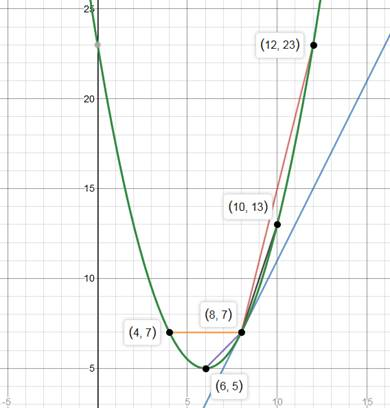Chapter 2.1, Problem 16E

Chapter
Section
Textbook Problem
1 views

# The displacement (in feet) of a particle moving in a straight line is given by s = 1 2 t 2 − 6 t + 23 , where t is measured in seconds.(a) Find the average velocity over each time interval:(i) [4, 8](ii) [6, 8](iii) [8, 10](iv) [8, 12](b) Find the instantaneous velocity when. t = 8 .(c) Draw the graph of s as a function of t and draw the secant lines whose slopes are the average velocities in part (a). Then draw the tangent line whose slope is the instantaneous velocity in part (b).

To determine

a)To find::

The average velocity over time interval

Explanation

1) Formula:

The average velocity over a time interval [a,b] is given

Average velocity=sb-s(a)b-a

where s(t) is the displacement function.

2) Given:

s=12t2-6t+23.

3) Calculation:

i) The average velocity over time interval [4, 8]

Substitute the values of a = 4 and b = 8 in the average velocity formula, then

Average velocity=s8-s48-4

=1282-68+23-1242-64+234

=32-48+23-8+24-234

=04=0

ii) The average velocity over time interval [6, 8]

Substitute the values of a = 6 and b = 8 in the average velocity formula. Then we have

Average velocity=s8-s68-6

=1282-68+23-1262-66+232

=32-48+23-18+36-232

=22=1

iii) The average velocity over time interval [8, 10]

Substitute the values of a = 8 and b = 10 in the average velocity formula

To determine

(b)

To find::

The instantaneous velocity when t=8.

To determine

(c)

To plot:

The graph of s as a function of t and draw the secant lines whose slopes are the average velocities in part (a). Then draw the tangent line whose slope is theinstantaneous velocity in part (b).

Explanation:The green curve is the displacement curve. The blue line is the tangent line whose slope in instantaneous velocity. The others are secant lines.

### Still sussing out bartleby?

Check out a sample textbook solution.

See a sample solution

#### The Solution to Your Study Problems

Bartleby provides explanations to thousands of textbook problems written by our experts, many with advanced degrees!

Get Started

#### Find more solutions based on key concepts